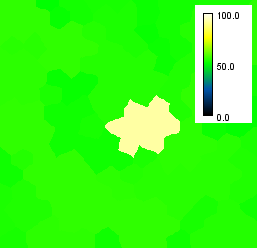# CLIJ2GPU accelerated image processing for everyone

CLIJ2 home

# Filtering between touching neighbors

Author: Robert Haase, May 2021

Source

This macro shows how to apply an get a voronoi image of a binary image in the GPU.

Clean up first and get test data: An image showing simulated tissue and another image with measurements for every cell where one cell has an increased value.

```run("Close All");

open("C:/structure/code/clij2-docs/src/main/resources/tissue.tif");
label_image = getTitle();
open("C:/structure/code/clij2-docs/src/main/resources/measurements.tif");
measurements_image = getTitle();
configure_visualization();
```

Init GPU and data

```run("CLIJ2 Macro Extensions", "cl_device=");
Ext.CLIJ2_clear();

// push data to GPU
Ext.CLIJ2_push(label_image);
Ext.CLIJ2_push(measurements_image);
```

## Visualizing local properties

As cells in tissues communicate with each other via membranes, we can measure regional properties of cells across their boundaries. For example local maximum of the given measurement.

```radius = 1;
ignore_background = true;
show(local_maximum_map);
```## Types of neighborhoods

### Touching neighbors

You can use differen types for neighborhoods: touching neighbors, n-nearest neighbors and proximal neighbors.

When producing maps of measurements of touching neighbors, the radius parameter can be used to define

• no neigbors (radius = 0),
• direct neighbors (radius = 1),
• neighbors of neighbors (radius = 2) and
• neighbors of neighbors of neighbors (radius = 3) to be taken into account for summarizing measurements.
```for (radius = 0; radius < 4; radius += 1) {
show(local_maximum_map);
}
```

### N nearest neighbors

Similarily, n-nearest neighbors can be used to describe a neighborhood:

```for (n = 10; n <= 30; n += 10) {
Ext.CLIJ2_maximumOfNNearestNeighborsMap(measurements_image, label_image, local_maximum_map, n);
show(local_maximum_map);
}
```

Note, when determining the local maximum, the neighborhood of the target pixel of the signal is relevant, not the source. Thus, unintuitive results can appear. Furthermore, per definition n=0 delivers the original parametric image:

```for (n = 0; n < 3; n += 1) {
Ext.CLIJ2_maximumOfNNearestNeighborsMap(measurements_image, label_image, local_maximum_map, n);
show(local_maximum_map);
}
```

### Proximal neighbors

You can also select neighbors according to the distance between their centroids. Also here, a distamce d=0 leads to the original parametric image as ouput.

```for (d = 0; d <= 50; d += 10) {
min_distance = 0;
max_distance = d;
Ext.CLIJ2_maximumOfProximalNeighborsMap(measurements_image, label_image, local_maximum_map, min_distance, max_distance);
show(local_maximum_map);
}

```

Clean up by the end.

```Ext.CLIJ2_clear();
```

These are just convenience functions used further up.

```function configure_visualization() {
run("Green Fire Blue");
setMinAndMax(0, 100);
run("Calibration Bar...", "location=[Upper Right] fill=White label=Black number=3 decimal=1 font=12 zoom=0.8 overlay");
}
function show(image) {
Ext.CLIJ2_pull(image);
configure_visualization();
}
```